### 用 Python 对数据进行相关性分析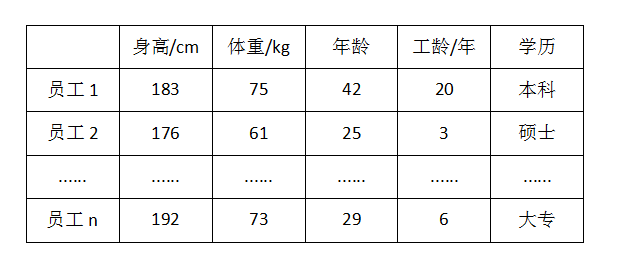``import seaborn as snsdata = sns.load_dataset('iris')df = data.iloc[:, :4] #取前四列数据``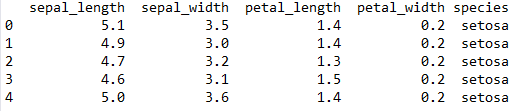``import numpy as npX = df['sepal_length']Y = df['petal_length']result1 = np.corrcoef(X, Y)````````result2 = np.corrcoef(df, rowvar=False)
``````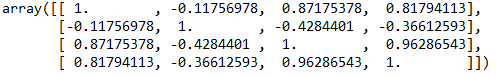``import scipy.stats as ssresult3 = ss.pearsonr(X, Y)``

``result4 = X.corr(Y)result5 = df.corr()``

result4结果是0.8717537758865833，result5结果如图5所示。这两个结果和前面所得的结果相同。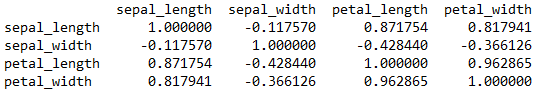``sns.pairplot(df)sns.pairplot(df , hue ='sepal_width');``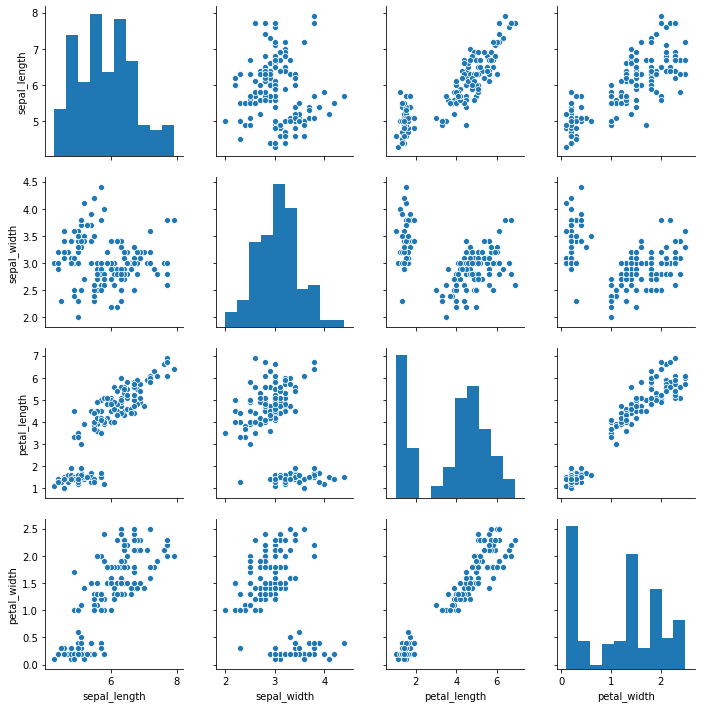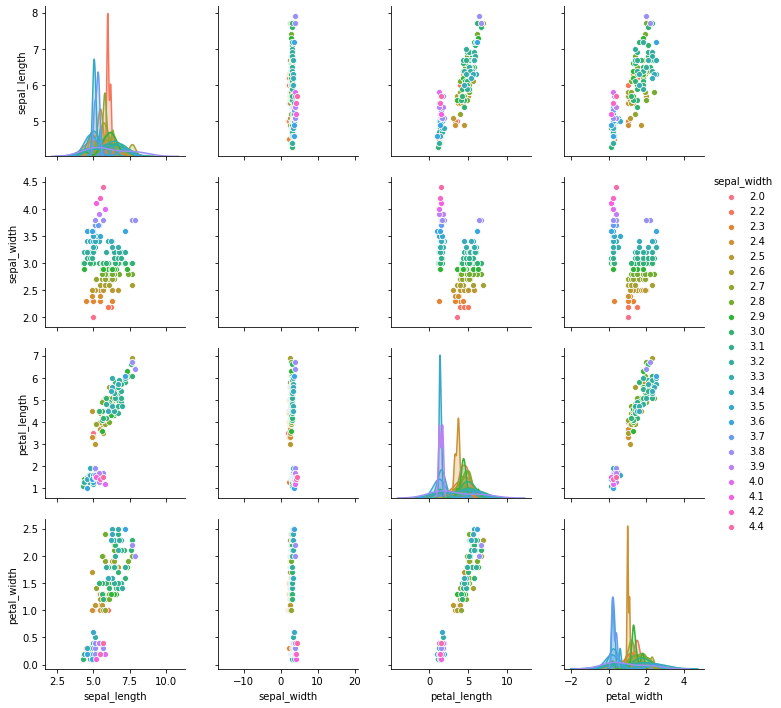``import pandas as pdpd.plotting.scatter_matrix(df, figsize=(12,12),range_padding=0.5);``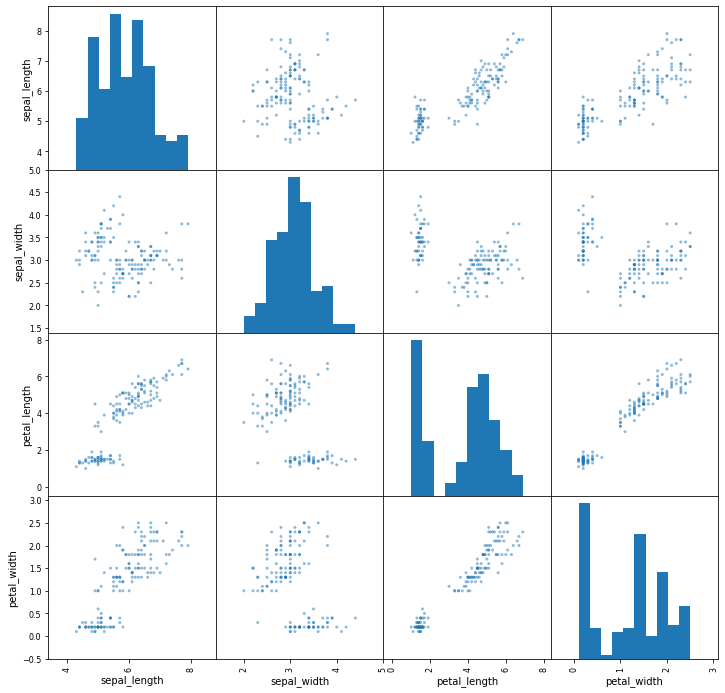``import matplotlib.pyplot as pltfigure, ax = plt.subplots(figsize=(12, 12))sns.heatmap(df.corr(), square=True, annot=True, ax=ax)``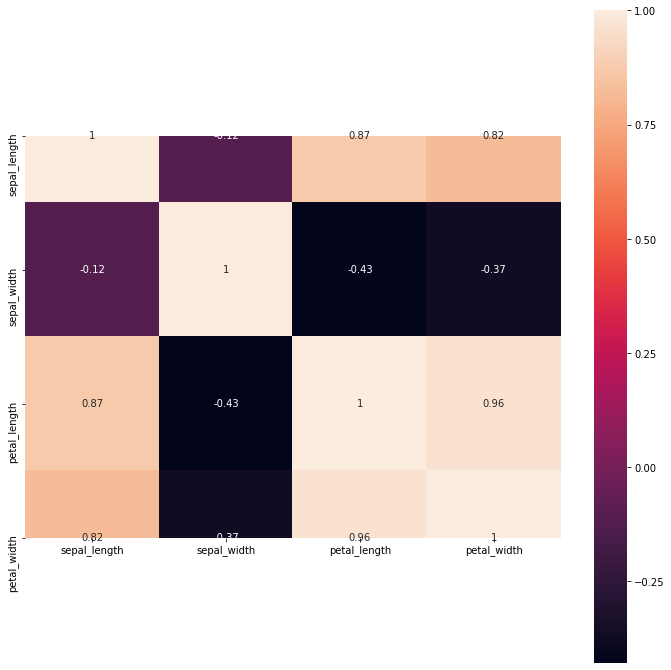Python中文社区作为一个去中心化的全球技术社区，以成为全球20万Python中文开发者的精神部落为愿景，目前覆盖各大主流媒体和协作平台，与阿里、腾讯、百度、微软、亚马逊、开源中国、CSDN等业界知名公司和技术社区建立了广泛的联系，拥有来自十多个国家和地区数万名登记会员，会员来自以工信部、清华大学、北京大学、北京邮电大学、中国人民银行、中科院、中金、华为、BAT、谷歌、微软等为代表的政府机关、科研单位、金融机构以及海内外知名公司，全平台近20万开发者关注。5 分钟解读 Python 中的链式调用▼点击成为社区会员   喜欢就点个在看吧